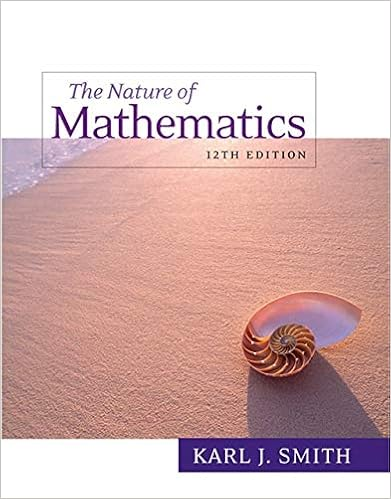# XM1SOL - MAT 126 Calculus B Fall 2009 Midterm I Solutions 1...

• Notes
• 4
• 100% (1) 1 out of 1 people found this document helpful

This preview shows page 1 - 2 out of 4 pages.

##### We have textbook solutions for you!
The document you are viewing contains questions related to this textbook.The document you are viewing contains questions related to this textbook.
Chapter 18 / Exercise 13
Nature of Mathematics
SmithExpert Verified
MAT 126: Calculus B, Fall 2009Midterm I Solutions1.Evaluate the integral by interpreting it as an area:Z31f(x)dx,wheref(x) =(2-xif 1x2x-2if 2x3
2.Evaluatelimn-→∞1nnXj=111 +(jn)2by interpreting the limit as an integral, and using the Evaluation Theorem.
##### We have textbook solutions for you!
The document you are viewing contains questions related to this textbook.The document you are viewing contains questions related to this textbook.
Chapter 18 / Exercise 13
Nature of Mathematics
SmithExpert Verified
•••